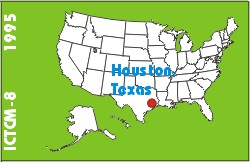# Calculus Remediation Using Databases

### Russell J. Hendel

POB 34662
USA
Phone: (215) 596-6830
Fax: (215) 596-5084

list of all papers by this author

 Click to access this paper: paper.txt

## ABSTRACT

This presentation will focus on a (typical) student problem: The 'WHERE DO I BEGIN,' problem. It will be shown that remediation can be accomplished using database theory.

Using Calculus II for purposes of illustration we may more specifically define the problem as follows: There are many students who KNOW how to implement the various integration techniques---parts, partial fractions, trigonometric substitutions,.... In other words, these students COULD SOLVE a problem of the form 'Integrate the following function using the TECHNIQUE OF....'. However some of these students may be unable to solve a problem of the form'Integrate the following function.'

We may describe these students as being INTERMEDIATE REMEDIAL-- they are better than those students who cannot implement particular techniques but are inferior to those students who can do problems without being prodded on which technique to use.

A characteristic symptom of INTERMEDIATE REMEDIALS is the typical response, 'WHERE DO I BEGIN.' However, once informed of the right technique these students can do the problems.

Having defined the problem we proceed to discuss the solution. For purposes of illustration consider a problem requesting integration of a rational function. It is reasonable to assume that a good student goes thru a mental list of integration techniques and selects that technique which is used when the integrand is a rational function (partial fractions). Our goal then is to give the intermediate remedial student the same skill.

Let us describe in further detail how the good student selects the technique of partial fractions. Imagine a database table of techniques. The first column of each row is labeled with a TECHNIQUE NAME--parts, partial fractions... The second column of each row is labeled with an INTEGRAND FORM---product of exponential and powers, rational functions etc. The remaining columns in each row give the STEPS by which the particular TECHNIQUE is implemented. (The full paper contains the database)

The good student seeks that row whose integrand-form column has 'rational function.' This results in the row beginning with partial fractions. THE CRUCIAL POINT IS THAT THE GOOD STUDENT HAS IN EFFECT PERFORMED THE FOLLOWING query ON THE technique DATABASE: 'SELECT * FROM techniques WHERE integrand = rational function.' It follows that one possible approach to helping the intermediate remedial is to teach him/her to use the TECHNIQUE database.

Keyword(s): pedagogy, software